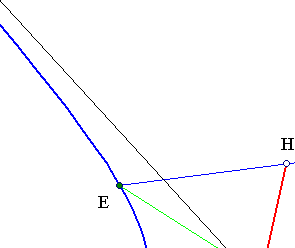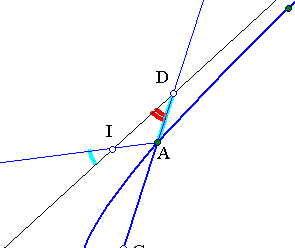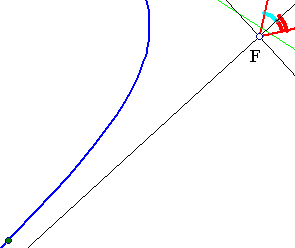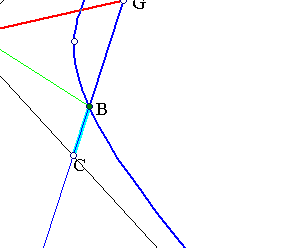##Rectangular Hyperbola Asymptotic Property

Consider a rectangular hyperbola (its asymptotic lines are orthogonal) and three points A, B, E on it. Then the lines FG, FH, joining the center F of the hyperbola with the middles of AB, AE respectively, make an angle at F equal to angle(BAE) or its complementary. One can formulate this also in the following way: the angle of two lines (a,b) is equal to the angle of their conjugates (a',b') with respect to a rectangular hyperbola or its complementary.
In particular, if B, E are symmetric w.r. to the center F of the hyperbola, then HFGA is a parallelogram and the asymptotic lines are bisectors of the angle(GFH).In fact, from the orthogonality at F and the equality of DA, BC (see HyperbolaAsymptoticProperty.html ) follows that FGD and FHI are isosceli triangles.
For an interesting application of this property to triangles with vertices on a rectangular hyperbola see the file OrthoRectangular.html .